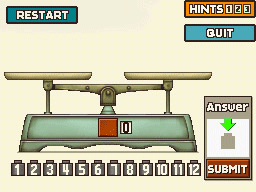### 131 Heavier or Lighter?Layton's Challenges
The Golden Apple's House

Puzzle 131Hint 1Hint 2Hint 3SolutionStart with Weighing 1

Weighing 1Weigh 1 2 3 4 on the left and 5 6 7 8 on the right

If the weights are even go to Weighing 2a
If the left side is lighter go to Weighing 2b
If the right side is lighter go to Weighing 2c

Weighing 2aWeigh 1 2 3 on the left and 9 10 11 on the right

If the weights are even then weight 12 is the answer
If the left side is lighter go to Weighing 3a
If the right side is lighter go to Weighing 3b

Weighing 2bWeigh 1 2 9 on the left and 3 4 5 on the right

If the weights are even go to Weighing 3c
If the left side is lighter go to Weighing 3d
If the right side is lighter go to Weighing 3e

Weighing 2cWeigh 1 2 9 on the left and 3 4 5 on the right

If the weights are even go to Weighing 3f
If the left side is lighter go to Weighing 3g
If the right side is lighter go to Weighing 3h

Weighing 3aWeigh 9 on the left and 10 on the right

If the weights are even then weight 11 is the answer
If the left side is lighter then weight 10 is the answer
If the right side is lighter then weight 9 is the answer

Weighing 3bWeigh 9 on the left and 10 on the right

If the weights are even then weight 11 is the answer
If the left side is lighter then weight 9 is the answer
If the right side is lighter then weight 10 is the answer

Weighing 3cWeigh 6 on the left and 7 on the right

If the weights are even then weight 8 is the answer
If the left side is lighter then weight 7 is the answer
If the right side is lighter then weight 6 is the answer

Weighing 3dWeigh 1 5 on the left and 3 4 on the right

If the weights are even then weight 2 is the answer
If the left side is lighter then weight 1 is the answer
If the right side is lighter then weight 5 is the answer

Weighing 3eWeigh 3 on the left and 4 on the right

If the left side is lighter then weight 3 is the answer
If the right side is lighter then weight 4 is the answer

Weighing 3fWeigh 6 on the left and 7 on the right

If the weights are even then weight 8 is the answer
If the left side is lighter then weight 6 is the answer
If the right side is lighter then weight 7 is the answer

Weighing 3gWeigh 3 on the left and 4 on the right

If the left side is lighter then weight 4 is the answer
If the right side is lighter then weight 3 is the answer

Weighing 3hWeigh 1 5 on the left and 3 4 on the right

If the weights are even then weight 2 is the answer
If the left side is lighter then weight 5 is the answer
If the right side is lighter then weight 1 is the answer

Submit TipDrag the lightest weight to the answer box before tapping submit

Progress4983 Picarats and 200 Hint Coins (US)
4973 Picarats and 200 Hint Coins (UK)

130 Too Many Queens 5